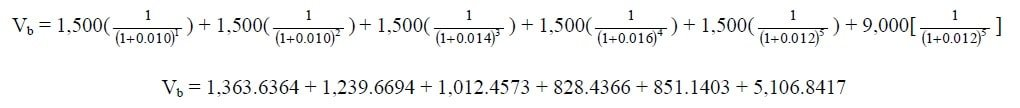Select Page

Bond Discounting Problems and Solutions

Problem 1:

A \$100 par value bond bearing a coupon rate of 12 percent will mature after 5 years. What is the value of the bond, if the discount rate is 15 percent by factor formula and table?

Solution:

Vb = 12 (PVIFA 15%, 5) + 100 (PVIF 15%, 5)

Vb = 12 (3.3522) + 100 (0.4972)

Problem 2:

\$100 par value debenture paying coupon of \$10 per annum is redeemed at par in 3 years. The average annual discount rate is 6%. Coupons are paid semi annually, what is the price of this debt by general floating equation?

Problem 3:

\$10,000 zero coupon bonds is redeemed at par in 4 years. The average annual discount rate is 7%. What is the price of this bond?

Problem 4:

The par value of 10% debenture is \$1,000 with maturity is 3 years. What would be the price by general floating formula if interest rate is (a) 12%, (b) 10% and (c) 8%?

Problem 5:

A bond has a par value of \$100, a coupon rate of 10.75% and matures in 5 years. If interest is paid annually and the required rate of return is 10%, what is the bond’s value by general constant rate formula?

Problem 6:

\$1,000 bond, 9%, due 4/1/2022, with interest each April 1 and October 1, issued 4/1/2002. Calculate intrinsic value of debt assuming market rate of return is 10 %?

Problem 7:

Irredeemable bond paying coupon of \$10 per annum has an average annual discount rate 6%. Coupons are paid semi-annually, what is the price of this bond?

Problem 8:

8% corporate bond is due in 10 years. What is the price of the bond if the nominal yield to maturity is 12% p.a. using factor formula? (Note that the bond pays the coupons semiannually and has a face value equal to \$5,000)?

Solution:

Vb = 400/2 (PVIFA12%/2, 10*2) + 5,000(PVIF12%/2, 10*2)

Vb = 200 (11.470) + 5,000 (0.3118)

Vb = 2,294 + 1,559

Problem 9:

You have a \$1,000 par, 5% coupon, Pak Treasury bond with 7 years remaining in its life. Coupons are paid semiannually and the next coupon payment is exactly six months away. The market interest rate is 6% (nominal rate with semiannual discounting). What is the current price of this bonds assuming that 10 bonds held to maturity?

Solution:

Vb = 500 (PVIFA 6%/2, 7*2) + 10,000 (PVIF 6%/2, 7*2)

Vb = 500 (11.296) + 10,000 (0.6611)

Vb = 5,648 + 6,611

Problem 10:

ABC is Public Limited Company. The company board of director was decided to offer 1,000 no. of bonds of par value of \$10 each in 2004; carrying 15 percent coupon rate and 5 year maturity period, bond would mature in 2009. The discount rate in first year (2005) was 10 percent. The rate was same in 2006. After that market rate of return had increased to 14 % in 2007. Under rising inflation and political instability the rate further jumped to 16 percent in 2008. We are in 2009 year and expected that market rate will remain 12 percent in this year and market value of bonds will be 9 per bond (1,000 bonds). What will be present value of bond?1.2.